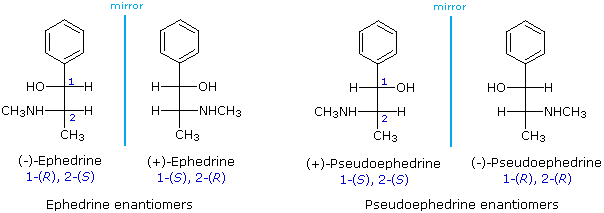# Fischer Projections

$$\newcommand{\vecs}{\overset { \rightharpoonup} {\mathbf{#1}} }$$ $$\newcommand{\vecd}{\overset{-\!-\!\rightharpoonup}{\vphantom{a}\smash {#1}}}$$$$\newcommand{\id}{\mathrm{id}}$$ $$\newcommand{\Span}{\mathrm{span}}$$ $$\newcommand{\kernel}{\mathrm{null}\,}$$ $$\newcommand{\range}{\mathrm{range}\,}$$ $$\newcommand{\RealPart}{\mathrm{Re}}$$ $$\newcommand{\ImaginaryPart}{\mathrm{Im}}$$ $$\newcommand{\Argument}{\mathrm{Arg}}$$ $$\newcommand{\norm}{\| #1 \|}$$ $$\newcommand{\inner}{\langle #1, #2 \rangle}$$ $$\newcommand{\Span}{\mathrm{span}}$$ $$\newcommand{\id}{\mathrm{id}}$$ $$\newcommand{\Span}{\mathrm{span}}$$ $$\newcommand{\kernel}{\mathrm{null}\,}$$ $$\newcommand{\range}{\mathrm{range}\,}$$ $$\newcommand{\RealPart}{\mathrm{Re}}$$ $$\newcommand{\ImaginaryPart}{\mathrm{Im}}$$ $$\newcommand{\Argument}{\mathrm{Arg}}$$ $$\newcommand{\norm}{\| #1 \|}$$ $$\newcommand{\inner}{\langle #1, #2 \rangle}$$ $$\newcommand{\Span}{\mathrm{span}}$$$$\newcommand{\AA}{\unicode[.8,0]{x212B}}$$

The problem of drawing three-dimensional configurations on a two-dimensional surface, such as a piece of paper, has been a long-standing concern of chemists. The wedge and hatched line notations we have been using are effective, but can be troublesome when applied to compounds having many chiral centers. As part of his Nobel Prize-winning research on carbohydrates, the great German chemist Emil Fischer, devised a simple notation that is still widely used. In a Fischer projection drawing, the four bonds to a chiral carbon make a cross with the carbon atom at the intersection of the horizontal and vertical lines. The two horizontal bonds are directed toward the viewer (forward of the stereogenic carbon). The two vertical bonds are directed behind the central carbon (away from the viewer). Since this is not the usual way in which we have viewed such structures, the following diagram shows how a stereogenic carbon positioned in the common two-bonds-in-a-plane orientation ( x–C–y define the reference plane ) is rotated into the Fischer projection orientation (the far right formula). When writing Fischer projection formulas it is important to remember these conventions. Since the vertical bonds extend away from the viewer and the horizontal bonds toward the viewer, a Fischer structure may only be turned by 180º within the plane, thus maintaining this relationship. The structure must not be flipped over or rotated by 90º.In the above diagram, if x = CO2H, y = CH3, a = H & b = OH, the resulting formula describes (R)-(–)-lactic acid. The mirror-image formula, where x = CO2H, y = CH3, a = OH & b = H, would, of course, represent (S)-(+)-lactic acid.

Using the Fischer projection notation, the stereoisomers of 2-methylamino-1-phenylpropanol are drawn in the following manner. Note that it is customary to set the longest carbon chain as the vertical bond assembly.The usefulness of this notation to Fischer, in his carbohydrate studies, is evident in the following diagram. There are eight stereoisomers of 2,3,4,5-tetrahydroxypentanal, a group of compounds referred to as the aldopentoses. Since there are three chiral centers in this constitution, we should expect a maximum of 23 stereoisomers. These eight stereoisomers consist of four sets of enantiomers. If the configuration at C-4 is kept constant (R in the examples shown here), the four stereoisomers that result will be diastereomers. Fischer formulas for these isomers, which Fischer designated as the "D"-family, are shown in the diagram. Each of these compounds has an enantiomer, which is a member of the "L"-family so, as expected, there are eight stereoisomers in all. Determining whether a chiral carbon is R or S may seem difficult when using Fischer projections, but it is actually quite simple. If the lowest priority group (often a hydrogen) is on a vertical bond, the configuration is given directly from the relative positions of the three higher-ranked substituents. If the lowest priority group is on a horizontal bond, the positions of the remaining groups give the wrong answer (you are in looking at the configuration from the wrong side), so you simply reverse it.The aldopentose structures drawn above are all diastereomers. A more selective term, epimer, is used to designate diastereomers that differ in configuration at only one chiral center. Thus, ribose and arabinose are epimers at C-2, and arabinose and lyxose are epimers at C-3. However, arabinose and xylose are not epimers, since their configurations differ at both C-2 and C-3.

### Contributors

This page titled Fischer Projections is shared under a CC BY-NC-SA 4.0 license and was authored, remixed, and/or curated by William Reusch.